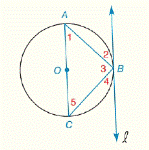Chapter 6.CR, Problem 11CR### Elementary Geometry for College St...

6th Edition
Daniel C. Alexander + 1 other
ISBN: 9781285195698

#### Solutions

Chapter
Section### Elementary Geometry for College St...

6th Edition
Daniel C. Alexander + 1 other
ISBN: 9781285195698
Textbook Problem
3 views

# G i v e n :           ⨀ O   w i t h   t a n g e n t   l   a n d   m ∠ 1 = 46 °           F i n d :             m ∠ 2 , m ∠ 3 ,     m ∠ 4 ,     m ∠ 5To determine

To find: m2, m3, m4, m5

Explanation

Theorem:

1) An angle inscribed in a semicircle is a right angle.

2) The measure of an angle formed by a tangent and a chord drawn to the point of tangency is one-half the measure of the intercepted arc.

3) The measure of an inscribed angle of a circle is one-half the measure of its intercepted arc.

Calculation:

Given that

m1=46° and l is tangent to the circle at point B.

By theorem, An angle inscribed in a semicircle is a right angle.

m3 is inscribed in a semicircle.

So, m3=90°.

Since, sum of the three angles in a triangle is 180°

In ABC, m1+m5+m3=180°

Let m1=46°,m3=90°

46°+m5+90°=180°

m5+136°=180°

Subtract 136°, m5+136°-136°=180°-136°

So, m5=44°

And m5=44° is the angle intercepted by the arc AB^

### Still sussing out bartleby?

Check out a sample textbook solution.

See a sample solution

#### The Solution to Your Study Problems

Bartleby provides explanations to thousands of textbook problems written by our experts, many with advanced degrees!

Get Started

#### Prove the identity. 55. sin1cos=csc+cot

Single Variable Calculus: Early Transcendentals, Volume I

#### In Exercises 29-34, rationalize the denominator of each expression. 29. 131

Applied Calculus for the Managerial, Life, and Social Sciences: A Brief Approach

#### Simplify: 1518

Elementary Technical Mathematics